# Short Answer Type Questions: Polynomials - Notes | Study Mathematics (Maths) Class 9 - Class 9

 1 Crore+ students have signed up on EduRev. Have you?

Q1. Write the numerical co-efficient and degree of each term of: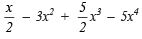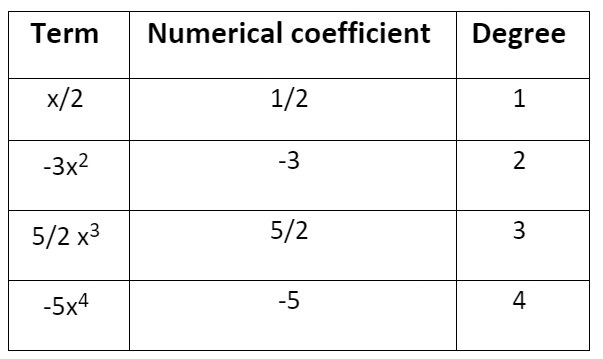Q2. Find the remainder when x3 – ax2 + 4x – a is divided by (x – a).

p(x) = x– ax2 + 4x – a
(x – a) = 0
⇒ x = a
∴ p(a) = (a)3 – a(a)2 + 4(a) – a = a3 – a+ 4a – a = 4a – a = 3a
∴ The required remainder = 3a.

Q3. When the polynomial kx3 + 9x2 + 4x – 8 is divided x + 3, then a remainder 7 is obtained.
Find the value of k.

Here, p(x) = kx3 + 9x+ 4x – 8
Since, Divisor = x + 3
∴ x + 3 = 0
⇒ x = –3
∴ p(–3) = 7
⇒ k(–3)3 + 9(–3)+ 4(–3) – 8 = 7
⇒ –27k + 81 – 12 – 8 = 7
⇒ –27k = 7 – 81 + 12 + 8
⇒ –27k = 27 – 81
⇒ –27k = –54
⇒ k= -(54/27) = 2
Thus, the required value of k = 2.

Q4. (a) For what value of k, the polynomial x2 + (4 – k)x + 2 is divisible by x – 2?
(b) For what value of ‘m’ is x3 – 2mx2 + 16 is divisible by (x + 2)?

(a) Here p(x) = x+ 4x – kx + 2
If p(x) is exactly divisible by x – 2, then p(2) = 0
i.e. (2)2 + 4(2) – k(2) + 2 = 0
⇒ 4 + 8 – 2k + 2 = 0
⇒ 14 – 2k = 0
⇒ 2k = 14
⇒ k= 14/2 = 7
Thus, the required value of k is 7.

(b) Here, p(x) = x3 – 2mx2 + 16
∴ p(–2) = (–2)3 –2(–2)2m + 16
⇒ –8 –8m + 16
⇒ –8m + 8
Since, p(x) is divisible by x + 2
∴ p(–2) = 0
or –8m + 8 = 0
⇒ m = 1

Q5. Factorize x2 – x – 12.

We have  x2 – x – 12
⇒ x– 4x + 3x – 12
⇒ x(x – 4) + 3(x – 4)
⇒ (x – 4)(x + 3)
Thus, x– x – 12 = (x – 4)(x + 3)

Q6. If x + (1/2)x  = 5, then find the value of x2 +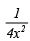We have x + (1/2)x  = 5
Squaring both sides, we get: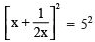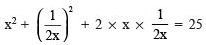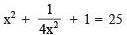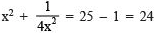Thus, the required value of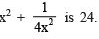Q7. Check whether (x – 1) is a factor of the polynomial x3 – 27x2 + 8x + 18.

Here, p(x) = x– 27x2 + 8x + 18, (x – 1) will be a factor of p(x) only if (x – 1) divides p(x) leaving a remainder 0.
For  x – 1 = 0
⇒ x = 1
∴ p(1) = (1)3 – 27(1)2 + 8(1) + 18
⇒ 1 – 27 + 8 + 18
⇒ 27 – 27
⇒ 0
Since, p(1) = 0
∴ (x – 1) is a factor of p(x).
Thus, (x – 1) is a factor of x3 – 27x2 + 8x + 18.

Q8. Find the remainder when f(x) =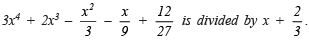Here f(x) =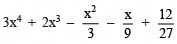Divisor = x+(2/3)
since, x+(2/3) = 0 ⇒ x = -2/3
∴ Remainder = f (-2/3)
i.e. Remainder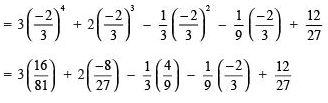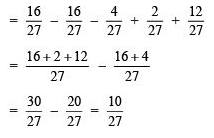Thus, the required remainder =  (10/27)

Q9. Find the value of k, if (x – k) is a factor of x6 – kx5 + x4 – kx3 + 3x – k + 4.

Here, p(x) = x6 – kx5 + x– kx3 + 3x – k + 4
If (x – k) is a factor of p(x), then p(k) = 0
i.e (k)6 – k(k5) + k4 – k(k3) + 3k – k + 4 = 0
⇒ k– k6 + k4 – k4 + 3k – k + 4 = 0
⇒ 2k + 4 = 0
⇒ 2k = – 4
⇒ k = (-4/2) = –2
Thus, the required value of k is –2.

Q10. Factorize: 9a2 – 9b2 + 6a + 1

9a2 – 9b2 + 6a + 1
⇒ [9a2 + 6a + 1] – 9b
⇒ [(3a)2 + 2(3a)(1) + (1)2] – (3b)
⇒ (3a + 1)2 – (3b)2
⇒ [(3a + 1) + 3b][(3a + 1) – 3b]    {using x– y2 = (x – y)(x + y)}
⇒ (3a + 1 + 3b)(3a + 1 – 3b)

Q11. Find the value of x3 + y3 – 12xy + 64, when x + y = –4.

x3 + y– 12xy + 64
⇒ (x)3 + (y)3 + (4)– 3(x)(y)(4)
⇒ [x2 + y2 + 42 – xy – y. 4 – 4 .  x](x + y + 4)
⇒ [x2 + y2 + 16 – xy – 4y – 4x][x + y + 4]                    ...(1)
Since, x + y = –4 ∴ x + y + 4 = 0                                 ...(2)
From (1) and (2), we have x3 + y– 12xy + 64
⇒ [x+ y2 + 16 – xy – 4y – 4x] = 0
Thus, x3 + y3 – 12xy + 64 = 0.

The document Short Answer Type Questions: Polynomials - Notes | Study Mathematics (Maths) Class 9 - Class 9 is a part of the Class 9 Course Mathematics (Maths) Class 9.
All you need of Class 9 at this link: Class 9

## Mathematics (Maths) Class 9

88 videos|397 docs|109 tests
 Use Code STAYHOME200 and get INR 200 additional OFF

## Mathematics (Maths) Class 9

88 videos|397 docs|109 tests

Track your progress, build streaks, highlight & save important lessons and more!

,

,

,

,

,

,

,

,

,

,

,

,

,

,

,

,

,

,

,

,

,

;